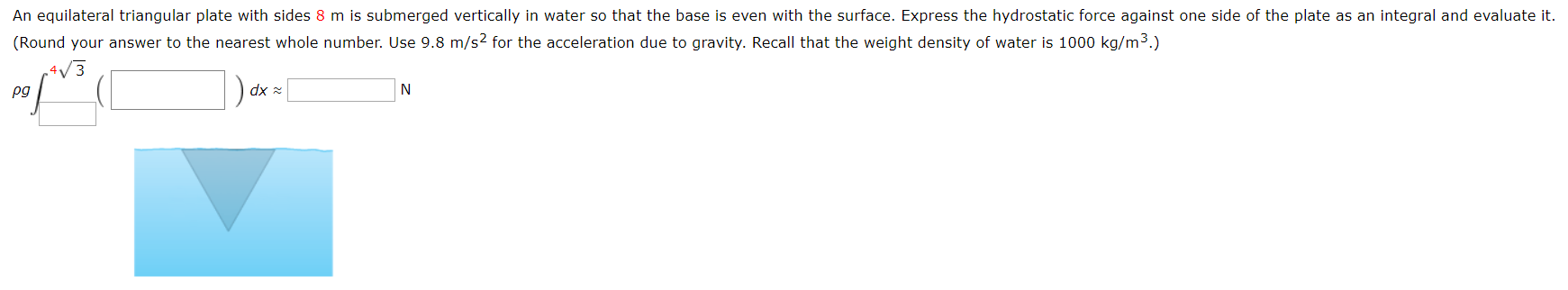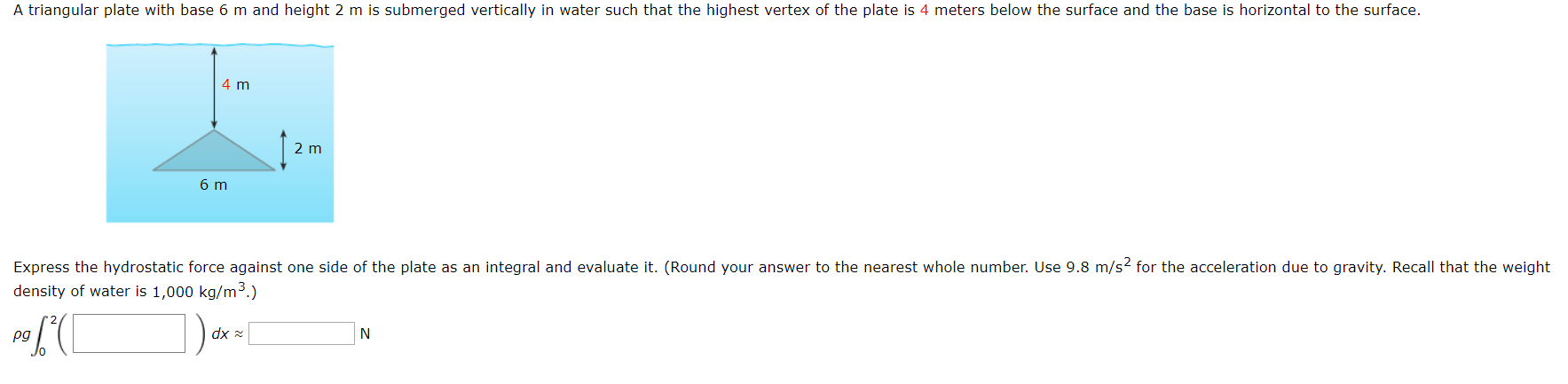# An equilateral triangular plate with sides 8 m is submerged vertically in water so that the base is even with the surface. Express the hydrostatic force against one side of the plate as an integral and evaluate it. (Round your answer to the nearest whole number. Use 9.8 m/s2 for the acceleration due to gravity. Recall that the weight density of water is 1000 kg/m3.) dx N pg A triangular plate with base 6 m and height 2 m is submerged vertically in water such that the highest vertex of the plate is 4 meters below the surface and the base is horizontal to the surface. 4 m 2 m 6 m Express the hydrostatic force against one side of the plate as an integral and evaluate it. (Round your answer to the nearest whole number. Use 9.8 m/s2 for the acceleration due to gravity. Recall that the weight density of water is 1,000 kg/m3.) dx N pg

Question

Solve parts these 2 parts of the question. Image attached.help_outlineImage TranscriptioncloseAn equilateral triangular plate with sides 8 m is submerged vertically in water so that the base is even with the surface. Express the hydrostatic force against one side of the plate as an integral and evaluate it. (Round your answer to the nearest whole number. Use 9.8 m/s2 for the acceleration due to gravity. Recall that the weight density of water is 1000 kg/m3.) dx N pg fullscreenhelp_outlineImage TranscriptioncloseA triangular plate with base 6 m and height 2 m is submerged vertically in water such that the highest vertex of the plate is 4 meters below the surface and the base is horizontal to the surface. 4 m 2 m 6 m Express the hydrostatic force against one side of the plate as an integral and evaluate it. (Round your answer to the nearest whole number. Use 9.8 m/s2 for the acceleration due to gravity. Recall that the weight density of water is 1,000 kg/m3.) dx N pg fullscreen

### Want to see this answer and more?

Experts are waiting 24/7 to provide step-by-step solutions in as fast as 30 minutes!*

*Response times vary by subject and question complexity. Median response time is 34 minutes and may be longer for new subjects.
Tagged in
MathCalculus

### Integration